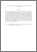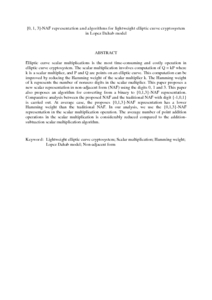# {0, 1, 3}-NAF representation and algorithms for lightweight elliptic curve cryptosystem in Lopez Dahab model

## Citation

Md. Yasin, Sharifah and Nor, Rozi Nor Haizan and Din, Jamilah and Muda, Zaiton (2014) {0, 1, 3}-NAF representation and algorithms for lightweight elliptic curve cryptosystem in Lopez Dahab model. International Review on Computers and Software, 9 (9). pp. 1541-1547. ISSN 1828-6003; ESSN: 1828-6011

## Abstract

Elliptic curve scalar multiplications is the most time-consuming and costly operation in elliptic curve cryptosystem. The scalar multiplication involves computation of Q = kP where k is a scalar multiplier, and P and Q are points on an elliptic curve. This computation can be improved by reducing the Hamming weight of the scalar multiplier k. The Hamming weight of k represents the number of nonzero digits in the scalar multiplier. This paper proposes a new scalar representation in non-adjacent form (NAF) using the digits 0, 1 and 3. This paper also proposes an algorithm for converting from a binary to {0,1,3}-NAF representation. Comparative analysis between the proposed NAF and the traditional NAF with digit {-1,0,1} is carried out. At average case, the proposes {0,1,3}-NAF representation has a lower Hamming weight than the traditional NAF. In our analysis, we use the {0,1,3}-NAF representation in the scalar multiplication operation. The average number of point addition operations in the scalar multiplication is considerably reduced compared to the addition-subtraction scalar multiplication algorithm.Preview
PDF (Abstract)
0.pdfView Item